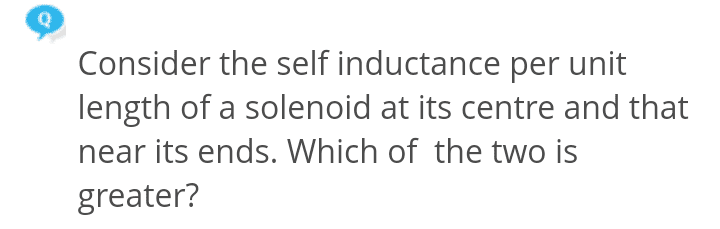# Self inductance of a solenoid

• Jahnavi
In summary, self induction per unit length signifies that the EMF of the inductor (=a solenoid is an inductor, and oftentimes the core is filled with iron to greatly increase the inductance), ## \mathcal{E}_{inductor}=-L \frac{dI}{dt} ##, is greater than the other EMF's.

## Homework Statement## The Attempt at a Solution

Self inductance L = μ0n2πr2l . This means it depends only on geometrical factors .

So , self inductance in both the cases should be equal .

But if I think in terms of L = Φ/I , then Magnetic field at the center is twice that at the ends (assuming long solenoid ) . In that case flux at the center will be twice that at the ends and so will be the self inductance .

I am not sure which of the above two reasoning is correct .

#### Attachments

The formula you have is for the self inductance of a very long solenoid. You are correct that the magnetic field is no longer uniform at the ends of a solenoid of finite length, and is approximately (almost exactly) 1/2 at the ends, and thereby the ## -\frac{d \Phi}{dt} ## will be one-half as much per unit length near the ends as anywhere near the middle. Since inductance ## L=\frac{\Phi}{I} ## the effect on the inductance in the region near the ends is that it contributes half as much per unit length. ## \\ ## Incidentally, one can take the deivative of the last equation and write that the EMF in the inductor is ## \mathcal{E}=-\frac{d \Phi}{dt}=-L \frac{dI}{dt} ##.

•Jahnavi
Do you mean SI at the center is greater ?

You are correct that the magnetic field is no longer uniform at the ends of a solenoid of finite length, and is approximately (almost exactly) 1/2 at the ends,

I think this is for a long solenoid , not a finite length solenoid .

The magnetic field of a long solenoid is ## B_z=n \mu_o I ## throughout its entire length and is very uniform with ## B_r=B_{\phi}=0 ##. ## \\ ## It is a very well-known (or somewhat well-known) problem from advanced E&M theory that the magnetic field ## B_z ## at the openings of the solenoid is ## B_z=\frac{1}{2}n \mu_o I ##. ## \\ ## For the details: This result is because the magnetic field of a magnetized cylinder (with the same dimensions as the solenoid) with uniform magnetization ## M_z ## in the z-direction has the exact same magnetic field pattern as the solenoid, (both inside and outside the cylinder), with ## B_z= M_z ## inside and anywhere near the middle region. Some very straightforward calculations using the magnetic pole method give the result that there are surface magnetic charge densities ## \sigma_m=\vec{M} \cdot \hat{n} ## at the endfaces, resulting in a contribution of ## B_z=-(1/2) M_z ## inside the cylinder near this "plane of surface charge", (comes from ## H_z=-\frac{\sigma}{2 \mu_o} ## inside the cylinder, near the endface, with an inverse square law fall-off as one moves away from the endface), and ## \vec{B}=\mu_o \vec{H}+\vec{M} ##. (Note: This result is quite basic for understanding the concept of magnetic surface concepts in the magnetic surface current methodology for computing the magnetic field of a permanent magnet. Notice how the magnetic surface current per unit length ## \vec{K}_m=\vec{M} \times \hat{n}/\mu_o ## corresponds to the solenoid current per unit length of ## nI ##).## \\ ## In any case, this is a well-known result that is presented in advanced E&M courses, and so the result can be used without going through all of the details. And your second line of reasoning in the OP is the correct one.

Last edited:
•Jahnavi
OK .Do you agree that , considering energy density = (1/2)B20 and , with the similar reasoning as in the OP , energy density at the center is greater ?

#### Attachments

•Jahnavi said:
OK .

View attachment 226658

Do you agree that , considering energy density = (1/2)B20 and , with the similar reasoning as in the OP , energy density at the center is greater ?
That is correct.•Jahnavi
Thanks .

Could you explain qualitatively what does self induction per unit length signify ? I mean what does it tell you about the solenoid ? Why per unit length ?

Jahnavi said:
Thanks .

Could you explain qualitatively what does self induction per unit length signify ? I mean what does it tell you about the solenoid ?
In the differential equation for the basic series L-R-C circuit, you can write ## \mathcal{E}=L \frac{dI}{dt}+IR+\frac{Q}{C} ##, where ## \mathcal{E} ## are any additional EMF's. The EMF of the inductor (=a solenoid is an inductor, and oftentimes the core is filled with iron to greatly increase the inductance), ## \mathcal{E}_{inductor}=-L \frac{dI}{dt} ##, so we put it on the other side of the equation with a plus sign. And then the current ## I=\frac{dQ}{dt } ##, so the equation is a second order differential equation in ## Q ## . ## \\ ## A solenoid is often used to create a nearly uniform magnetic field, as with an MRI machine, but it can also be used to create an inductive circuit element. In the laboratory, we had small inductors with iron filled cores, (about 1" long and 1/4" in diameter), and I seem to recall they had an inductance of ## L \approx 100 \, \mu H ##. ## \\ ## The concept of inductance per unit length could be useful if you are trying to design an inductor, but near the ends, as this problem is showing, the effective length, and correspondingly the inductance, is reduced somewhat from what the formula gives.

Last edited:
•Jahnavi